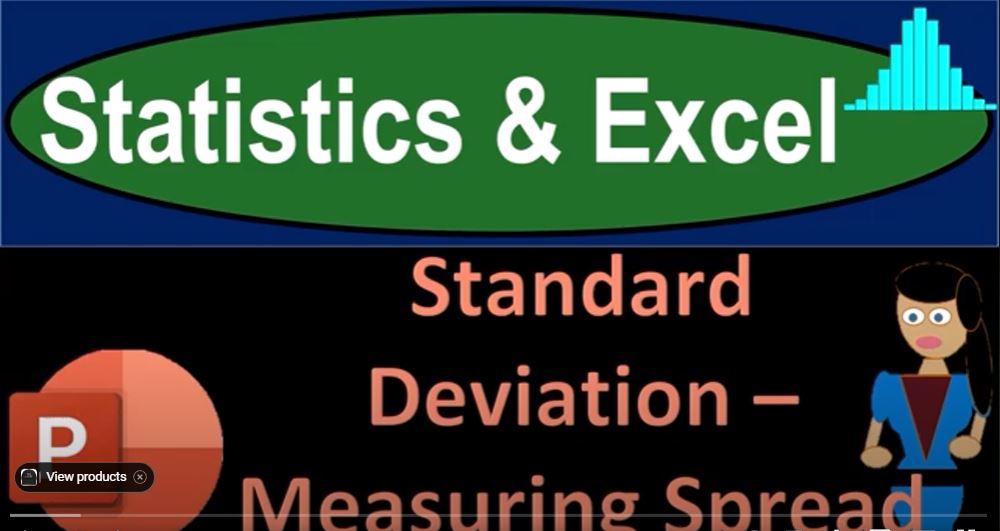## Standard Deviation – Measuring Spread 1406 Statistics & Excel

https://youtu.be/vvJtA570bIwf

In the world of statistics and data analysis, our primary mission is to confront the challenge of taking a list of numbers and structuring them in a way that offers meaning.

This involves summarizing data using numerical and pictorial tools. In this blog, we’ll focus on measures of data dispersion or spread, building upon our understanding of central tendencies like the mean and median. Specifically, we’ll delve into the standard deviation and variance, crucial tools for quantifying data spread.

Measuring Central Tendency:

Before delving into measures of data spread, let’s briefly recap central tendencies:

1. Mean (Average): The sum of all data divided by the number of data items. Denoted as x̄ (x-bar) or μ (mu), it represents the balance point of the data. Prone to the influence of outliers.
2. Median: The middle number in an ordered list of data. Resilient against the impact of outliers.

Understanding Dispersion and the Five-Number Summary:

To understand data spread, we often use the five-number summary, which includes the smallest point, first quartile, median, third quartile, and maximum. While this summary offers a rough idea of data spread, it has limitations. To gain a more detailed understanding, we introduce variance and standard deviation.

Variance and Standard Deviation:

1. Variance (S² or σ²): Represents the average of the squared differences between data points and the mean. It quantifies how spread out the numbers are from the mean. Variances are abstract but useful for comparing datasets.
2. Standard Deviation (S or σ): It’s the square root of the variance, giving the average distance of data points from the mean. Larger standard deviations indicate wider data spreads, while smaller values suggest data points are closer to the mean. Both variance and standard deviation are affected by outliers.

Why Squaring the Differences?

One might wonder why we square the differences instead of using absolute values. Squaring removes the negativity but also ensures that the population mean uniquely minimizes the sum of squared differences. It’s a mathematical necessity for this purpose.

Implications and Applications:

Statistics tools like variance and standard deviation are valuable for comparing data dispersion across different contexts. However, they must be interpreted within the context they represent to draw meaningful conclusions. Context is crucial when inferring meaning from statistics.

Summary:

In summary, while mean and median provide insight into central tendencies, they don’t reveal data spread. Histograms and the five-number summary offer visual insights but lack numerical summaries. Standard deviation and variance are numerical measures that quantify data spread. They may seem abstract but are essential for comparing datasets. Remember that context is key when interpreting statistics, and these tools are valuable for drawing meaningful conclusions from data.

In the upcoming sections, we’ll work through practical examples to deepen our understanding of these concepts.